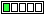All about flooble | fun stuff | Get a free chatterbox | Free JavaScript | Avatarsperplexus dot infoVive la petite différence! (Posted on 2015-04-06)Puzzle: If you choose an answer to this puzzle at random, what is the probability of a correct guess?

a) 25%
b) 50%
c) 60%
d) 25%

1. Explain why the above puzzle is a paradox.
2. By introducing a single decimal point in one of the choices - can you convert this paradox into a solvable problem?

 No Solution Yet Submitted by Ady TZIDON No RatingComments: ( Back to comment list | You must be logged in to post comments.)Possible Paradox Depending...| Comment 2 of 3 |There is a paradox present in the problem statement, of course depending on how it is interpreted in this case:

First if we assume, that there is one and only one UNIQUE pre-determined answer to the question (i.e either a,b,c,d) then of course we have p = 25%. This is of course, trivial, but is based on the symbol that represents the value. So although 'a' and 'd' represent the same identical value, they are unique symbols. If we were to throw them into a hat, you could only choose one, with equal probability for each.

However, if we consider the answer values themselves the situation is different. We would of course have p = 0.50 for the 25% answer, and p = 0.25 for the remaining answers of 60% and 50%. Over a long enough iteration, the answer of 25% will be chosen at random by 50% of people, and the remaining answers of 60% and 50% will be chosen by 25% of the population.

Now, herein lies the paradox (as soon as we start guessing answers at random):

Case 1: assume answer choice of 25% = True (correct answer)
If the answer choice of 25% is the correct answer, then only 25% of the population should have chosen it, NOT 50%.

Cases 2 & 3 : assume answer choice 50% = True
assume answer choice 60% = True

we yield the same conclusion, creating a logical paradox, since we would have only 25% of the population choosing 60% and 25% choosing 50% as the correct answer.

In each case you get a paradox, and ultimately it appears that there is no correct answer or guess!

We have a self referential system because the answer that is chosen at random, affects the answer to the problem itself!

By changing one of the 25% answers to say 2.5 or even 25.1% the paradox is resolved. There is a p= 0.25 for each possible answer choice. In this case, the only solution that will be logically consistent is 25%. If 25% of the population choose the answer of 25%, we have our solution! NO other possibility works

 Posted by alex on 2015-08-26 11:45:01Please log in:

 Search: Search body:
Forums (0)